# Less than sign

Here you will learn about the less than sign including the symbol used to represent it, and how to compare numbers and expressions using the less than sign.

Students will first learn about the less than sign as part of the number and operations in base 10 and number and operations – fractions in elementary school.

## What is the less than sign?

The less than sign is a mathematical symbol used to compare numbers and expressions.

It is one of the symbols used for inequalities along with the greater than symbol, >.

When stacking identical blocks, the height of 1 block is less than the height of 3 blocks. The lines joining the stacks give the shape and direction of the inequality symbols.

The less than sign is,

The wide end of the symbol always faces the bigger number or expression – the symbol looks open towards the bigger number and ‘points’ at the smaller value like an arrow.

For example,

This is read as ‘4 is less than 9’.

The less than sign is used to compare;

Whole numbers 12<15 This is read as ‘12 is less than 15’.
Decimals 1.3<2.5 This is read as ‘1.3 is less than 2.5’.
Fractions \cfrac{1}{4}<\cfrac{1}{3} This is read as ‘one fourth is less than one third’.

### What is the less than sign?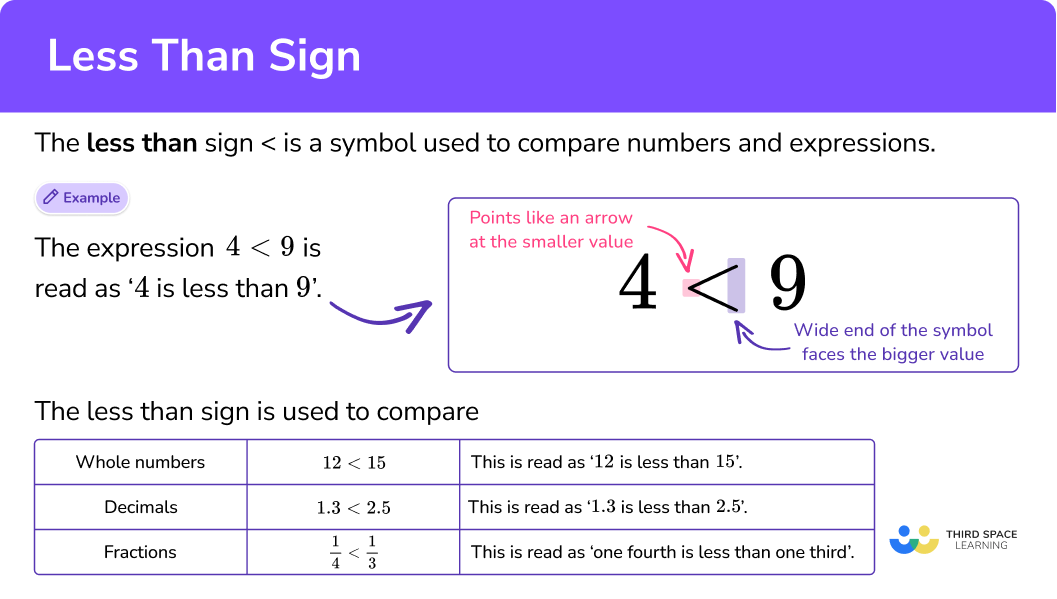## Common Core State Standards

• Grade 1: Number and Operations in Base Ten (1.NBT.B.3)
Compare two two-digit numbers based on meanings of the tens and ones digits, recording the results of comparisons with the symbols >, \, =, and <.

• Grade 2: Number and Operations in Base Ten (2.NBT.A.4)
Compare two three-digit numbers based on meanings of the hundreds, tens, and ones digits, using >, \, =, and < symbols to record the results of comparisons.

• Grade 3: Number and Operations – Fractions (3.NF.A.3d)
Compare two fractions with the same numerator or the same denominator by reasoning about their size. Recognize that comparisons are valid only when the two fractions refer to the same whole. Record the results of comparisons with the symbols >, \, =, or <, and justify the conclusions, for example, by using a visual fraction model.

• Grade 4: Number and Operations in Base Ten (4.NBT.A.2)
Read and write multi-digit whole numbers using base-ten numerals, number names, and expanded form. Compare two multi-digit numbers based on meanings of the digits in each place, using >, \, =, and < symbols to record the results of comparisons.

• Grade 4: Number and Operations – Fractions (4.NF.A.2)
Compare two fractions with different numerators and different denominators, for example, by creating common denominators or numerators, or by comparing to a benchmark fraction such as \frac{1}{2}. Recognize that comparisons are valid only when the two fractions refer to the same whole. Record the results of comparisons with symbols > , \, =, or <, and justify the conclusions, for example, by using a visual fraction model.

• Grade 5: Number and Operations in Base Ten (5.NBT.3b)
Compare two decimals to thousandths based on meanings of the digits in each place, using >, \, =, and < symbols to record the results of comparisons.

## How to compare values using the less than sign

In order to compare values using the less than sign:

1. Write the given numbers into a place value chart.
2. Starting with the greatest place value, compare the digits of the number, until you find digits that are different.
3. Write the values with the correct symbol, or place the numbers on the correct sides of a given symbol.

## Less than sign examples

### Example 1: comparing two-digit numbers

Write the correct sign, > or <, in the box.

1. Write the given numbers into a place value chart.

2Starting with the greatest place value, compare the digits of the number, until you find digits that are different.

Looking at the place value chart, start comparing the greatest place value, the tens place.

37 has 3 tens and 45 has 4 tens. 3 tens is less than 4 tens, therefore, 37 is the smaller number.

3Write the values with the correct symbol, or place the numbers on the correct sides of a given symbol.

37 is less than 45.

Place the wide end of the symbol towards the 45, with the point facing the smaller number, 37.

### Example 2: comparing three-digit numbers

Write the correct sign, > or <, in the box.

Write the given numbers into a place value chart.

Starting with the greatest place value, compare the digits of the number, until you find digits that are different.

Write the values with the correct symbol, or place the numbers on the correct sides of a given symbol.

### Example 3: comparing multi-digit numbers

Write the correct sign, > or <, in the box.

Write the given numbers into a place value chart.

Starting with the greatest place value, compare the digits of the number, until you find digits that are different.

Write the values with the correct symbol, or place the numbers on the correct sides of a given symbol.

### Example 4: comparing decimals

Write the correct sign, > or <, in the box.

Write the given numbers into a place value chart.

Starting with the greatest place value, compare the digits of the number, until you find digits that are different.

Write the values with the correct symbol, or place the numbers on the correct sides of a given symbol.

## How to compare fractions using the less than sign

In order to compare fractions using the less than sign:

1. See if the fractions have equal numerators or denominators.
2. Use equivalent fractions to find a common denominator if needed.
3. Compare the fractions and write the answer using the original fractions and correct comparison symbol.

### Example 5: comparing fractions with same numerator

Which is smaller, \, \cfrac{2}{6} \, or \, \cfrac{2}{4} \, ? Write your answer using the less than sign.

See if the fractions have equal numerators or denominators.

Use equivalent fractions to find a common denominator if needed.

Compare the fractions and write the answer using the original fractions and correct comparison symbol.

### Example 6: comparing fractions using common denominator

Which is smaller, \, \cfrac{3}{5} \, or \, \cfrac{5}{10} \, ? Write your answer using the less than sign.

See if the fractions have equal numerators or denominators.

Use equivalent fractions to find a common denominator if needed.

Compare the fractions and write the answer using the original fractions and correct comparison symbol.

### Example 7: comparing fractions using common denominator

Which is smaller, \, \cfrac{2}{3} \, or \, \cfrac{3}{5} \, ? Write your answer using the less than sign.

See if the fractions have equal numerators or denominators.

Use equivalent fractions to find a common denominator if needed.

Compare the fractions and write the answer using the original fractions and correct comparison symbol.

### Teaching tips for less than sign

• Younger primary school students may find it funny to draw an alligator’s mouth, but this often leads to students focusing only on the bigger number rather than the entire comparison. It is important to highlight the meaning of the math symbols each time they are used and to have students use them as intended.

• When kids are starting to compare numbers, it’s crucial to give them the freedom to experiment hands-on. Manipulatives and use of a number line are an excellent approach to further the meaning for struggling students in higher grade levels. As students have a better knowledge of what it means to compare numbers, there will be time for worksheets as practice.

• When comparing numbers, it’s critical for learners to understand what each symbol represents. When students are learning the meaning of these symbols, they can be displayed in the classroom on an anchor chart or in interactive notebooks.

### Easy mistakes to make

• Confusing the less than and greater than symbols
The biggest mistake is writing the symbols the wrong way round. Remember, the sign should point at the smallest number like an arrow.
For example, 15 \, > \, 8, \, ‘15 is greater than 8’, or 8 \, < \, 15, \, ‘8 is less than 15’.

• Trying to compare fractions without common numerator or denominator
When comparing fractions, the fractions should have either a common numerator or denominator. You may have to convert them into equivalent fractions with common denominators, so you can compare the numerators.

This less than sign topic guide is part of our series on inequalities. You may find it helpful to start with the main inequalities topic guide for a summary of what to expect or use the step-by-step guides below for further detail on individual topics. Other topic guides in this series include:

### Practice less than sign questions

1. Which is smaller, 41 or 47? Write your answer using the less than comparison symbol.

41 > 4741 < 4747 > 4147 < 41Write the numbers you are comparing into a place value chart.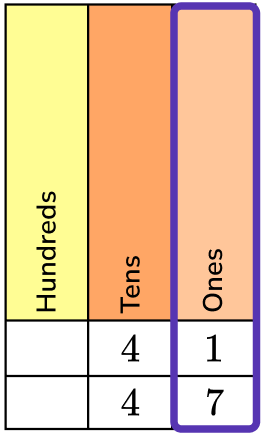Start with the largest place value chart, the tens, and compare the digits.

The digits in the tens place are the same, so move to the ones place to compare.

41 has 1 one and 47 has 7 ones.

1 one is less than 7 ones.

41 is less than 47.

41 < 47.

2. Which is smaller, 729 or 792? Write your answer using the less than comparison symbol.

729 < 792792 > 729729 > 792792 < 729Write the two numbers into a place value chart.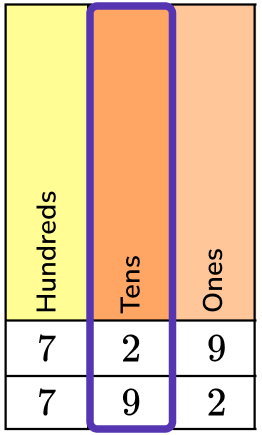Start with the largest place value chart, the hundreds place, and compare the digits.

The digits in the hundreds place are the same, so move to the tens place to compare.

729 has 2 tens and 792 has 9 tens.

2 tens is less than 9 tens.

729 is less than 792.

729 < 792.

3. Which is smaller, 42,087 or 42,187? Write your answer using the less than comparison symbol.

42,187 < 42,08742,187 > 42,08742,087 > 42,18742,087 < 42,187Write the two numbers into a place value chart.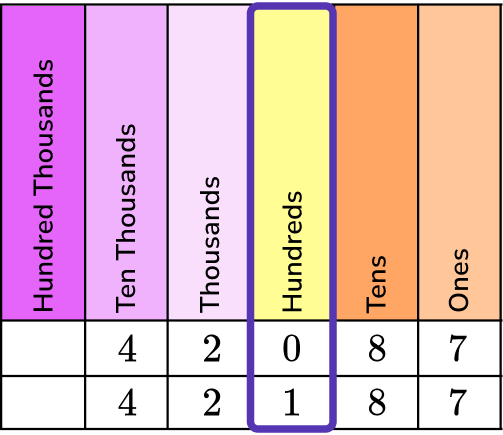Start with the largest place value chart, the ten thousands place, and compare the digits.

The digits in the ten thousands place and thousands place are the same, so move to the hundreds place to compare.

42,087 has 0 hundreds and 42,198 has 1 hundred.

0 hundreds is less than 1 hundred.

42,087 is less than 42,187.

42,087 < 42,187.

4. Which is smaller, 3.002 or 3.02? Write your answer using the less than comparison symbol.

3.002 > 3.023.02 < 3.0023.002 < 3.023.02 > 3.002Write the two numbers into a place value chart.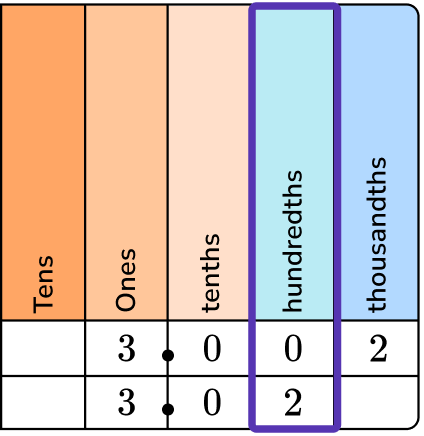Start with the largest place value chart, the ones place, and compare the digits.

The digits in the ones place and the tenths place are the same, so move to the hundredths place to compare.

3.002 has 0 hundredths and 3.02 has 2 hundredths.

0 hundredths is less than 2 hundredths.

3.002 is less than 3.02.

3.002 < 3.02.

5. Which is smaller, \, \cfrac{4}{11} \, or \, \cfrac{4}{9} \, ? Write your answer using the less than comparison symbol.

\cfrac{4}{11} \, > \, \cfrac{4}{9}\cfrac{4}{11} \, < \, \cfrac{4}{9}\cfrac{4}{9} \, > \, \cfrac{4}{11}\cfrac{4}{9} \, < \, \cfrac{4}{11}The fractions have equal numerators, so you do not need to make equivalent fractions.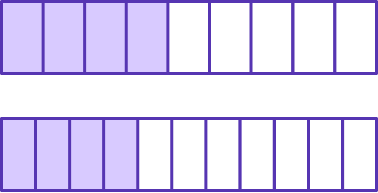Elevenths are smaller, so 4 elevenths will be less than 4 ninths.

Therefore, \, \cfrac{4}{11} \, is smaller than \, \cfrac{4}{9} \, .

\cfrac{4}{11} \, < \, \cfrac{4}{9} \, .

6. Which is smaller, \, \cfrac{2}{4} \, or \, \cfrac{5}{8} \, ? Write your answer using the less than comparison symbol.

\cfrac{5}{8} \, > \, \cfrac{2}{4}\cfrac{2}{4} \, > \, \cfrac{5}{8}\cfrac{5}{8} \, < \, \cfrac{2}{4}\cfrac{2}{4} \, < \, \cfrac{5}{8}The fractions do not have the same numerators (top numbers) or denominators (bottom numbers).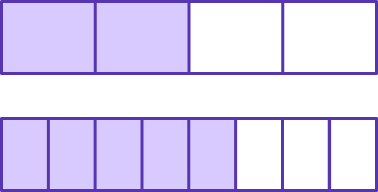To create a common denominator, double the fraction \, \cfrac{2}{4} \, to make the common denominator of 8.

\cfrac{2}{4}=\cfrac{2 \, \times \, 2}{4 \, \times \, 2}=\cfrac{4}{8}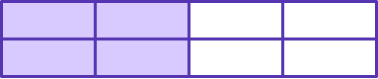Compare the fractions with common denominators \, \cfrac{4}{8} \, and \, \cfrac{5}{8} \, .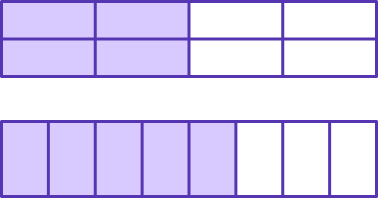\cfrac{4}{8} \, has 4 parts shaded in and \, \cfrac{5}{8} \, has 5 parts shaded in.

Since the parts are the same size, \, \cfrac{4}{8} \, is smaller.

\cfrac{2}{4} \, is smaller than \, \cfrac{5}{8} \, .

\cfrac{2}{4} \, < \, \cfrac{5}{8} \, .

## Less than sign FAQs

What’s the difference between the greater than and less than sign?

When using the greater than sign, the first number will be the smallest number followed by the second number being the larger number. The two numbers will be separated by the less than sign, <.

What other inequality symbols are there besides the greater than sign?

When comparing numbers, you will also use the less than sign, <, the equal sign, =, the less than or equal to sign, and the greater than or equal to sign, ≥ .

## Still stuck?

At Third Space Learning, we specialize in helping teachers and school leaders to provide personalized math support for more of their students through high-quality, online one-on-one math tutoring delivered by subject experts.

Each week, our tutors support thousands of students who are at risk of not meeting their grade-level expectations, and help accelerate their progress and boost their confidence.